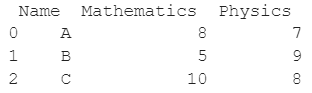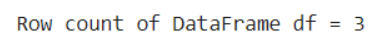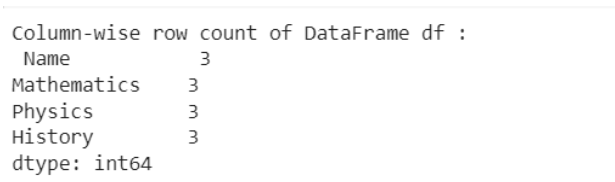# How Do I Get The Row Count Of A Pandas DataFrame?

A DataFrame is the primary data structure of the Pandas library and is commonly used for storing and working with tabular data. A common operation that could be performed on such data is getting the row count of an existing DataFrame in order to gauge exactly how many data points we have.

To start working with Pandas, we first need to import this statement in the Python code:

### Python 3 Code :

``import pandas as pd``

## Running Example

Let us understand this operation with the help of an example. Consider the following DataFrame containing 3 students with names ‘A’, ‘B’ and ‘C’ and their corresponding marks (out of 10) for two subjects, Mathematics and Physics.Code snippet for generating the above DataFrame is:

### Python 3 Code :

``````import pandas as pd

# Dictionary for our data
data = {'Name' : ['A', 'B', 'C'], 'Mathematics' : [8, 5, 10], 'Physics' : [7, 9, 8]}

# DataFrame for the dictionary
df = pd.DataFrame(data)

# Printing the DataFrame
print(df)``````

Here, data is a dictionary we created to initialize the DataFrame. For this, we use the DataFrame() function of the Pandas library which takes the dictionary as an argument and returns the required DataFrame.

Now, let’s say we need to find the row count of this DataFrame df, which is equal to the total number of students here. As seen in the DataFrame, the number of rows is 3.

Therefore, the expected output for the above example is 3.

Expected Output :Let us look at different ways of performing this operation :

## Get row count using the DataFrame.index property in Pandas

In this method, we shall use the index property of a Pandas DataFrame. The DataFrame.index property returns a Pandas Index object containing the label of the rows.

By using the len() function with the Pandas Index object returned by the DataFrame.index property as the parameter, we can find the number of entries in the Index object, which is equal to the row count of the DataFrame being considered.

### Python 3 Code :

``````import pandas as pd

# Dictionary for our data
data = {'Name' : ['A', 'B', 'C'], 'Mathematics' : [8, 5, 10], 'Physics' : [7, 9, 8]}

# DataFrame for the dictionary
df = pd.DataFrame(data)

# Getting row count of the DataFrame df
row_count = len(df.index)

# Printing the row count
print("Row count of DataFrame df =", row_count)``````

Output :## Get row count using the len() function in Pandas

In this method, we use the len() function to get the row count of a Pandas DataFrame.

When a Pandas DataFrame is passed as a parameter to the len() function, it returns the number of rows in the DataFrame, i.e. the row count.

### Python 3 Code :

``````import pandas as pd

# Dictionary for our data
data = {'Name' : ['A', 'B', 'C'], 'Mathematics' : [8, 5, 10], 'Physics' : [7, 9, 8]}

# DataFrame for the dictionary
df = pd.DataFrame(data)

# Getting row count of the DataFrame df
row_count = len(df)

# Printing the row count
print("Row count of DataFrame df =", row_count)``````

Output :## Get row count using the DataFrame.shape property in Pandas

In this method, we use the DataFrame.shape property to get the row count of a Pandas DataFrame. This property returns a tuple representing the dimensionality of the DataFrame.

The first element of the tuple represents the number of rows whereas the second element represents the number of columns of the DataFrame.

Therefore, the number of rows can be acquired using df.shape. This method gives us the number of rows of a DataFrame irrespective of the presence of NaN values.

### Python 3 Code :

``````import pandas as pd

# Dictionary for our data
data = {'Name' : ['A', 'B', 'C'], 'Mathematics' : [8, 5, 10], 'Physics' : [7, 9, 8]}

# DataFrame for the dictionary
df = pd.DataFrame(data)

# Getting row count of the DataFrame df
row_count = df.shape

# Printing the row count
print("Row count of DataFrame df =", row_count)``````

Output :## Get row count using the DataFrame.size property in Pandas

In this method, we use the DataFrame.size property to get the row count of a Pandas DataFrame.

The DataFrame.size property returns the total number of elements in the DataFrame, which is 9 here.

If we divide this number by the number of columns in the DataFrame, which is 3 here, we get the number of rows, since

``````number of elements = number of columns * number of rows

number of rows = number of elements / number of columns``````

The number of columns can be obtained using df.shape, similar to what has been described in method 3.

### Python 3 Code :

``````import pandas as pd

# Dictionary for our data
data = {'Name' : ['A', 'B', 'C'], 'Mathematics' : [8, 5, 10], 'Physics' : [7, 9, 8]}

# DataFrame for the dictionary
df = pd.DataFrame(data)

# Getting row count of the DataFrame df
row_count = df.size / df.shape

# Printing the row count
print("Row count of DataFrame df =", row_count)``````

Output :## Get row count using the DataFrame.pipe() function in Pandas

In this method, we use the DataFrame.pipe() function to get the row count of a Pandas DataFrame. We do this by passing len as a parameter to the function call, which is equivalent to using len(df).

The pipe function returns an object whose type is the same as the return type of the function that has been passed as a parameter, len here. This gives us the total row count of the DataFrame being considered.

### Python 3 Code :

``````import pandas as pd

# Dictionary for our data
data = {'Name' : ['A', 'B', 'C'], 'Mathematics' : [8, 5, 10], 'Physics' : [7, 9, 8]}

# DataFrame for the dictionary
df = pd.DataFrame(data)

# Getting row count of the DataFrame df
row_count = df.pipe(len)

# Printing the row count
print("Row count of DataFrame df =", row_count)``````

Output :## Get row count using the DataFrame.count() function in Pandas

In this method, we use the DataFrame.count() function to get the row count of a Pandas DataFrame. This function returns the number of non-NA entries for each column of the DataFrame.

In the example, being considered, there are no NA entries so the output of this function would be similar to the other methods.

### Python 3 Code :

``````import pandas as pd

# Dictionary for our data
data = {'Name' : ['A', 'B', 'C'], 'Mathematics' : [8, 5, 10], 'Physics' : [7, 9, 8]}

# DataFrame for the dictionary
df = pd.DataFrame(data)

# Getting row count of the DataFrame df
row_count = df.count()

# Printing the row count
print("Column-wise row count of DataFrame df :\n",row_count)``````

Output :## Get row count using the size and index property in Pandas

This method combines two approaches shown above, namely 1 and 4. The DataFrame.index property returns a Pandas Index object containing the label of the rows.

By using the size property on this object, we get the row count of the DataFrame being considered.

### Python 3 Code :

``````import pandas as pd

# Dictionary for our data
data = {'Name' : ['A', 'B', 'C'], 'Mathematics' : [8, 5, 10], 'Physics' : [7, 9, 8]}

# DataFrame for the dictionary
df = pd.DataFrame(data)

# Getting row count of the DataFrame df
row_count = df.index.size

# Printing the row count
print("Row count of DataFrame df =", row_count)``````

Output :## Conclusion

In this topic, we have learned to get the row count of an existing Pandas DataFrame, following a running example of test scores of students in different subjects, thus giving us an intuition of how this concept could be applied in real-world situations. Feel free to reach out to [email protected] in case of any suggestions.

Trending